SNVS690I January   2011  – August 2021

PRODUCTION DATA

1. Features
2. Applications
3. Description
4. Revision History
5. Pin Configuration and Functions
6. Specifications
7. Detailed Description
1. 7.1 Overview
2. 7.2 Functional Block Diagram
3. 7.3 Feature Description
4. 7.4 Device Functional Modes
8. Application and Implementation
1. 8.1 Application Information
2. 8.2 Typical Application
1. 8.2.1 Design Requirements
2. 8.2.2 Detailed Design Procedure
1. 8.2.2.1 Design Steps for the LMZ14201H Application
3. 8.2.3 Application Curve
9. Power Supply Recommendations
10. 10Layout
1. 10.1 Layout Guidelines
2. 10.2 Layout Example
11. 11Device and Documentation Support
12. 12Mechanical, Packaging, and Orderable Information

• NDW|7

## 6.6 Typical Characteristics

Unless otherwise specified, the following conditions apply: VIN = 24 V; Cin = 10-uF X7R Ceramic; CO = 47 uF; TA = 25°C.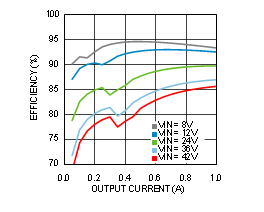Figure 6-1 Efficiency VOUT = 5 V, TA = 25°C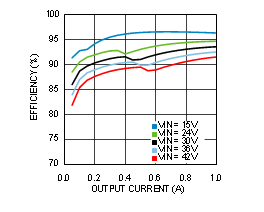Figure 6-3 Efficiency VOUT = 12 V, TA = 25°C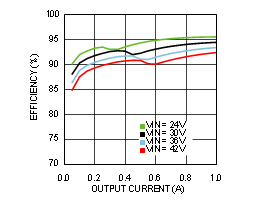Figure 6-5 Efficiency VOUT = 15 V, TA = 25°C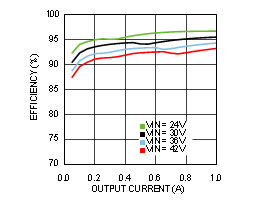Figure 6-7 Efficiency VOUT = 18 V, TA = 25°C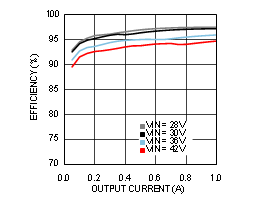Figure 6-9 Efficiency VOUT = 24 V, TA = 25°CFigure 6-11 Efficiency VOUT = 30 V, TA = 25°C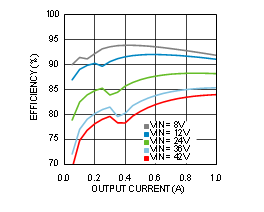Figure 6-13 Efficiency VOUT = 5 V, TA = 85°C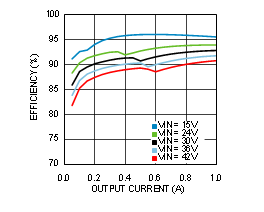Figure 6-15 Efficiency VOUT = 12 V, TA = 85°C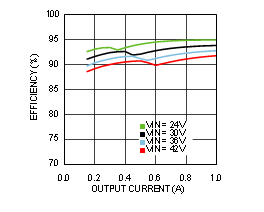Figure 6-17 Efficiency VOUT = 15 V, TA = 85°C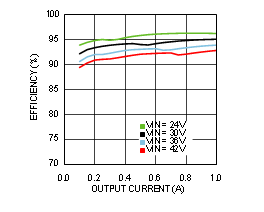Figure 6-19 Efficiency VOUT = 18 V, TA = 85°C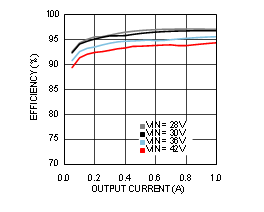Figure 6-21 Efficiency VOUT = 24 V, TA = 85°C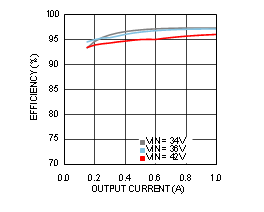Figure 6-23 Efficiency VOUT = 30 V, TA = 85°C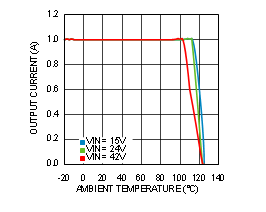Figure 6-25 Thermal Derating VOUT = 12 V, RθJA = 16°C/W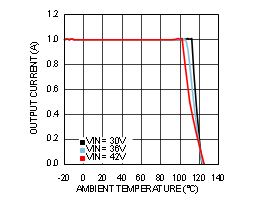Figure 6-27 Thermal Derating VOUT = 24 V, RθJA = 16°C/W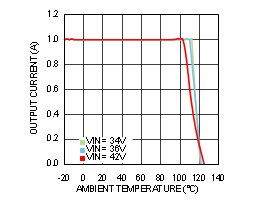Figure 6-29 Thermal Derating VOUT = 30 V, RθJA = 16°C/WFigure 6-31 Package Thermal Resistance RθJA 4 Layer PCB With 1-oz CopperFigure 6-33 Output Ripple VIN = 12 V, IOUT = 1 A, Ceramic COUT, BW = 200 MHz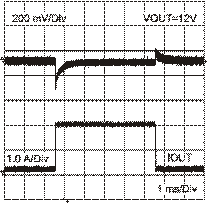Figure 6-35 Load Transient Response VIN = 24 V VOUT = 12 V Load Step from 10% to 100%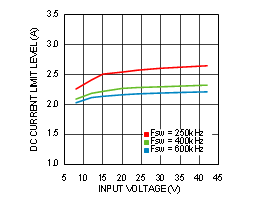Figure 6-37 Current Limit vs. Input Voltage VOUT = 5 V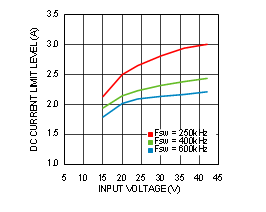Figure 6-39 Current Limit vs. Input Voltage VOUT = 12 V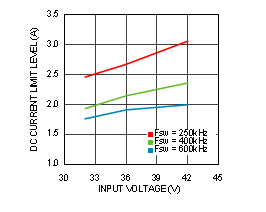Figure 6-41 Current Limit vs. Input Voltage VOUT = 24 V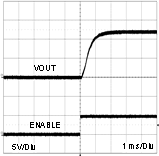Figure 6-43 Start-Up VIN = 24 V, IOUT = 1 AFigure 6-45 Conducted EMI, VOUT = 12 V Evaluation Board BOM and 3.3-µH, 1-µF LC Line Filter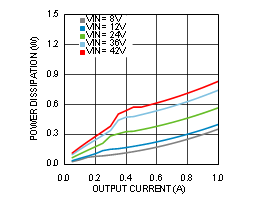Figure 6-2 Power Dissipation VOUT = 5 V, TA = 25°C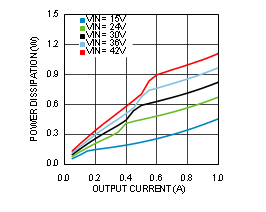Figure 6-4 Power Dissipation VOUT = 12 V, TA = 25°C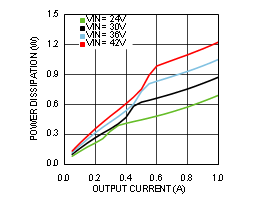Figure 6-6 Power Dissipation VOUT = 15 V, TA = 25°C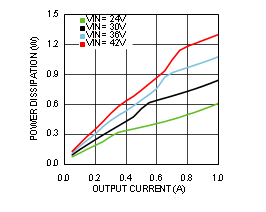Figure 6-8 Power Dissipation VOUT = 18 V, TA = 25°C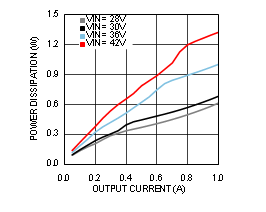Figure 6-10 Power Dissipation VOUT = 24 V, TA = 25°C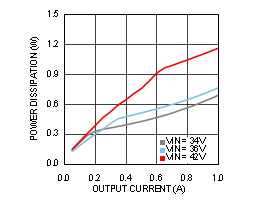Figure 6-12 Power Dissipation VOUT = 30 V, TA = 25°C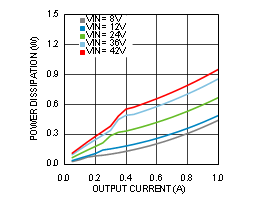Figure 6-14 Power Dissipation VOUT = 5 V, TA = 85°CFigure 6-16 Power Dissipation VOUT = 12 V, TA = 85°C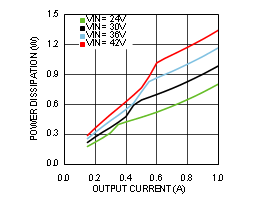Figure 6-18 Power Dissipation VOUT = 15 V, TA = 85°C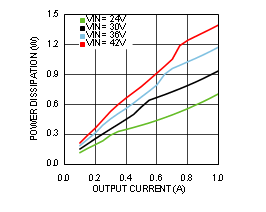Figure 6-20 Power Dissipation VOUT = 18 V, TA = 85°C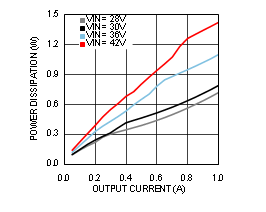Figure 6-22 Power Dissipation VOUT = 24 V, TA = 85°C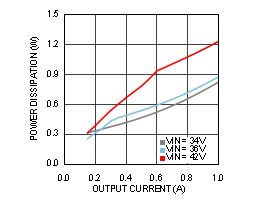Figure 6-24 Power Dissipation VOUT = 30 V, TA = 85°C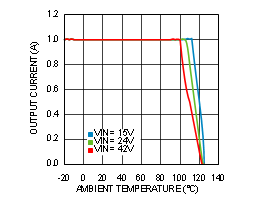Figure 6-26 Thermal Derating VOUT = 12 V, RθJA = 20°C/W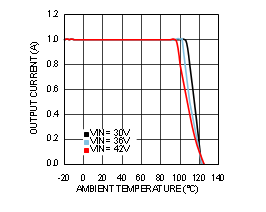Figure 6-28 Thermal Derating VOUT = 24 V, RθJA = 20°C/W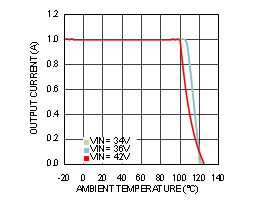Figure 6-30 Thermal Derating VOUT = 30 V, RθJA = 20°C/W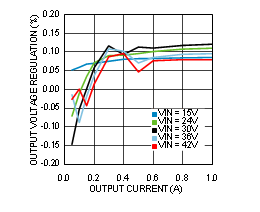Figure 6-32 Line and Load Regulation TA = 25°C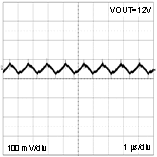Figure 6-34 Output Ripple VIN = 24 V, IOUT = 1 A, Polymer Electrolytic COUT, BW = 200 MHz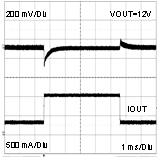Figure 6-36 Load Transient Response VIN = 24 V VOUT = 12 V Load Step From 30% to 100%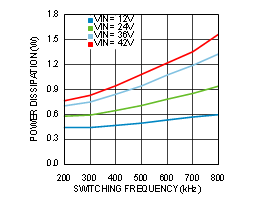Figure 6-38 Switching Frequency vs. Power Dissipation VOUT = 5 V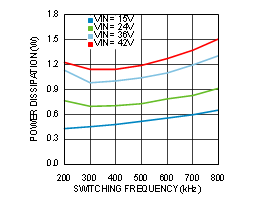Figure 6-40 Switching Frequency vs. Power Dissipation VOUT = 12 V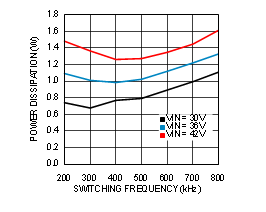Figure 6-42 Switching Frequency vs. Power Dissipation VOUT = 24 V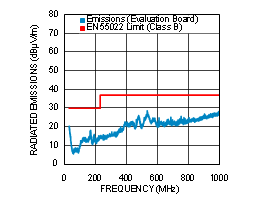Figure 6-44 Radiated EMI of Evaluation Board, VOUT = 12 V